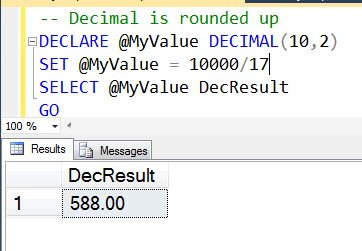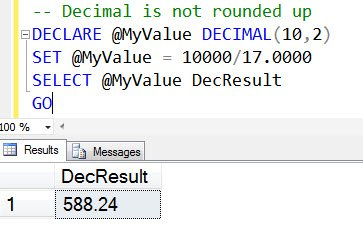# SQL SERVER – Puzzle – Why Decimal is Rounded Up?

Lots of people know the answer to this puzzle, but I am sure there are many who do not know the answer of the same as well. Here is the puzzle again for those who have vacation for the Holidays!

Here is the first script:

```-- Decimal is rounded up
DECLARE @MyValue DECIMAL(10,2)
SET @MyValue = 10000/17
SELECT @MyValue DecResult
GO
```

` `Here is the second script:

```-- Decimal is not rounded up
DECLARE @MyValue DECIMAL(10,2)
SET @MyValue = 10000/17.0000
SELECT @MyValue DecResult
GO
```Here is the question – When we execute the first script the decimal’s are rounded up and when we execute the second script the decimal’s are not rounded up. WHY?

Just leave your answer in the comments field. I will select two random winners in the first week of January and will send surprise gift worth USD 29.

Reference: Pinal Dave (https://blog.sqlauthority.com)

#### Related Posts

•Mukesh
December 30, 2014 12:29 pm

By default SQL will understand numeric value as integer.
In First scenario, 10000/17 means [Int/Int] and result is 588. But it assigned to decimal variable so value will display 588.00.
In Second scenario, 10000/17 means [Int/Decimal] so result will come in decimal and value will be 588.24. same value will assign to decimal variable and display 588.24 value in result.

•Arsalan Ahmed Khan
December 30, 2014 1:42 pm

ANS: Integer truncated decimal point value
=1000 / (17/17/17)
=1000 / (1/17)
[because of { 1/17 = 0.058823 } in decimal form number only in SQL it can write as ‘1.00/17’ or ‘1/17.00’ at least one(numerator/denominator) in decimal form but it is in the form of integer that’s why decimal point value truncated]
= 1000 / 0
->Divide by zero

•nivrutti
December 30, 2014 3:54 pm

Whenever there is division operation…
that time SQL checks condition that if denominator or numerator any one of it is decimal number then only we will get the un-rounded result.
If denominator and numerator both are integer numbers then we will get the rounded result only.
eg.
1) Select (1000/17)
Result : 58
2) Select (1000/17.0)
Result : 58.823529
3) Select (1000.00/17)
Result : 58.823529

•Gyan
December 30, 2014 7:13 pm

Hello Pinal,

Sql Server is very smart as if we perform operations for non decimal numbers it treated it as integers while if perform operation for decimal numbers it treated as float.
For example –
if the query is

SELECT 10000/(17/17/17.0) AS Result2

then result is – 170001.530013770123931115

it means whern we pick the inner part in select like
select 17/17/17.0 as InnerResult
then result is – 0.058823

and if we take
select 17/17/17 as InnerResult
then result is – 0

That is why the result of SELECT 10000/(17/17/17) AS Result2 gives the “Divide by zero error encountered.” error.

Best Regards,
Gyanendra Singh

•Hitesh Kumar
January 10, 2015 3:59 pm

if both dividend and divisor are integer than result will be integer. and if anyone or both from dividend and divisor is decimal than result will be decimal.

•Dharam
September 8, 2016 10:47 pm

First one is doing integer division that is it will give you integer only. Second one is doing decimal division which will give you decimal value

•Yashveer Gurjar
September 8, 2016 10:53 pm

The reason for the difference in the Outputs is :
1. The first Output is Integer as it the float is being divided by integer which will give a integer result.
2. The second output is a decimal because it is being divided with a float value which will result in a float output.

•Dpan
March 10, 2019 4:28 pm

DECLARE @MyValue DECIMAL(10,2),@divider decimal(10,2)
set @divider=17
SET @MyValue = 10000/@divider
SELECT @MyValue DecResult
GO

if you assaign the divider and output value as decimal , it will give decimal like above

DECLARE @MyValue DECIMAL(10,2),@divider int
set @divider=17
SET @MyValue = 10000/@divider
SELECT @MyValue DecResult
GO

if you assaign as integer , it will give integer op